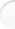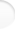Structure of Magic and Semi-Magic Squares, Methods and Tools for Enumeration by Francis GaspalouI give the main notations, definitions and conventions; it is not an exhaustive list.

 1. Notations

n Order of magic or semi-magic square

S Magic constant
S = n(n²+1)/2 for normal squares, made with numbers from 1 to n².
S = Σ + σ with:
Σ = A+B+C+... (capital letters)
σ = a+b+c+... (lower-case letters)

k Number of basis (a,b,c...;A,B,C...) in the decomposition of a square with capital and lower-case letters
k is a function of n

N Number of parameters
N=(n-1)² for semi-magic squares of order n
N=(n-1)²-2 for magic squares of order n
Note that the dimension of the vector space is N + 1 (one condition is given here: S is fixed in function of n; in other words, I deal with normal squares)

 A1 A2 A3 ... An B1 B2 B3 ... Bn C1 C2 C3 ... Cn ...
Cells of magic or semi-magic squares (or matrix of transformation)

 2. Definitions Top of the pageMagic square Rows, columns and the two diagonals are magic: the sum of numbers is constant (this sum is the "magic constant") Semi-magic square Rows and columns are magic, but not the two diagonals Pandiagonal square Rows, columns and broken diagonals are magic Semi-pandiagonal Specific square of order 4 (A2+B1+C4+D3=34 and A3+B4+C1+D2=34) Basis (a,b,c...;A,B,C...) Set of n values for lower-case letters a,b,c,... and of n values for capital letters A,B,C,.... allowing to build a table of addition Caution! It is different from the basis in a vector space. Intermediate square Square with capital and lower-case letters Isomorphic With the same structure Regular Each (capital or lower-case) letter occurs only one time in each line Semi-regular For each line, the sum of capital letters is Σ (and then the sum of lower-case letters is σ) Irregular For one line, the sum of capital letters is different from Σ (or the sum of lower-case letters is different from σ) Line Row, column or diagonal Latin square Square with n letters a, n letters b, etc. Each letter occurs only one time in each row and each column Gallic square Square with n letters a, n letters b, etc. It is not compulsory, as in a Latin square, that each row (or column) has only one a letter; it is sufficient that a occurs n times in the square. Idem for b, etc. Group Set of elements having special properties (in mathematics, you can define a rule which is associative, there is an identity element and each element has an inverse) Abstract definitionof a group Relations of definition of a group (relations on generators) Transposition Specific transformation made with permutations of rows and columns symmetrically located from the centre of the square

 3. Conventions Top of the pageI deal only with "normal" magic or semi-magic squares, i. e. with squares made with the numbers from 1 to n².

I don't divide by 8 the number of magic squares as it is usually done in the literature.
For example, I speak about 7 040 squares of order 4 and not about 7 040/8 = 880 squares.
As a matter of fact, for a given square, I think you have to consider all the variations of group G and not only the 8 classical variations (rotations and reflections) of octic group.

For counting the number of squares given by an intermediate square (square with capital and lower-case letters),
I don't take the transformations of this intermediate square.

Regular squares are for me a subset of semi-regular squares.